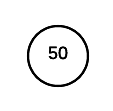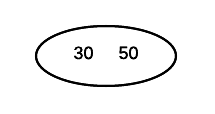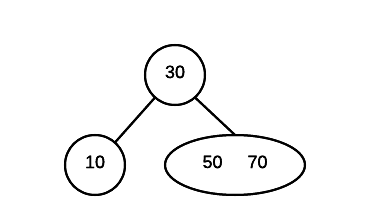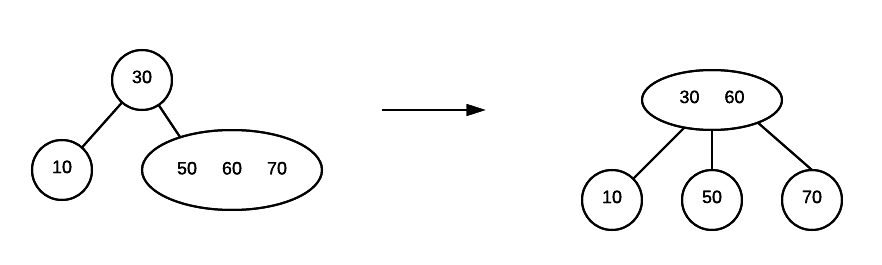# 2-3 Trees

A 2-3 Tree is a specific form of a B tree. A 2-3 tree is a search tree. However, it is very different from a binary search tree.

Here are the properties of a 2-3 tree:

1. each node has either one value or two value
2. a node with one value is either a leaf node or has exactly two children (non-null). Values in left subtree < value in node < values in right subtree
3. a node with two values is either a leaf node or has exactly three children (non-null). Values in left subtree < first value in node < values in middle subtree < second value in node < value in right subtree.
4. all leaf nodes are at the same level of the tree

## Insertion

The insertion algorithm into a two-three tree is quite different from the insertion algorithm into a binary search tree. In a two-three tree, the algorithm will be as follows:

1. If the tree is empty, create a node and put value into the node
2. Otherwise find the leaf node where the value belongs.
3. If the leaf node has only one value, put the new value into the node
4. If the leaf node has more than two values, split the node and promote the median of the three values to parent.
5. If the parent then has three values, continue to split and promote, forming a new root node if necessary

### Example:

Insert 50Insert 30Insert 10Insert 70Insert 60